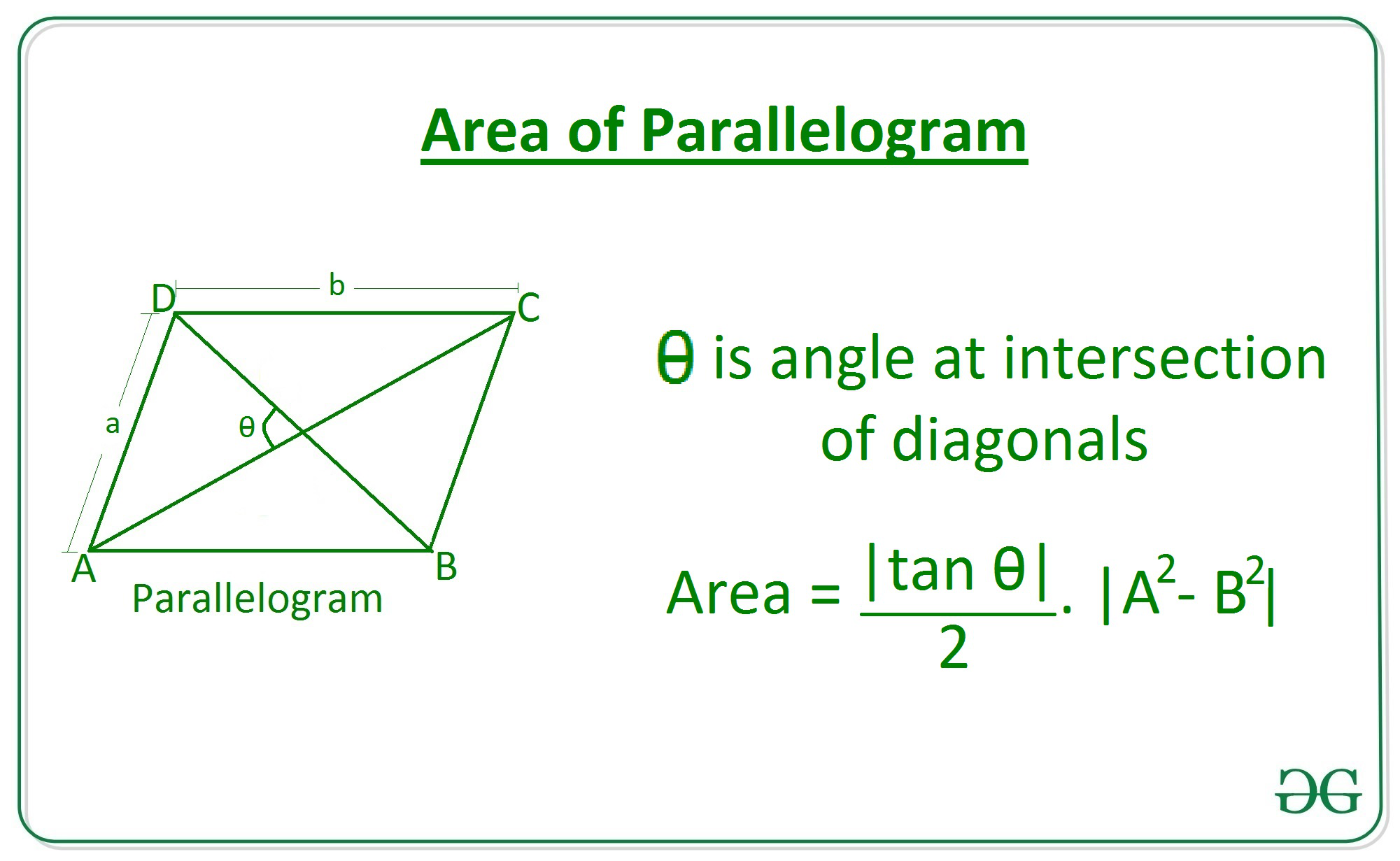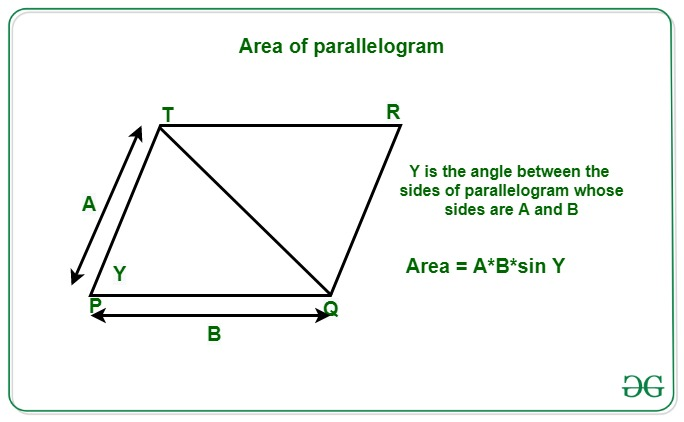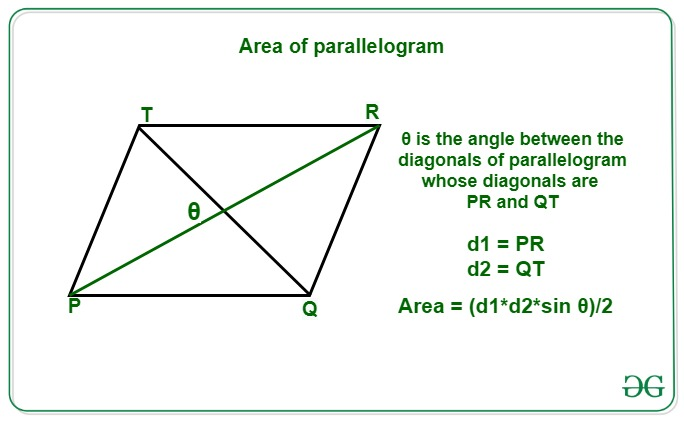# Program to calculate area of a parallelogram

Given integers A and B denoting the length of sides of a parallelogram and Y that is the angle between the sides and length of diagonals D1 and D2 of the parallelogram and an angle 0 at the intersection of the diagonal, the task is to find the area of the parallelogram from the information provided.

A parallelogram is a type of quadrilateral that has equal and parallel opposite sides and angle between them is not right angle.

Examples:

Input: A = 6, B = 10, 0 = 30
Output: 18.48
Explanation:
For the given sides 6 and 10 and for the angle 30 degree the area of parallelogram will be 18.48.

Input: A = 3, B = 5, Y = 45
Output: 10.61
Explanation:
For the given sides 3 and 5 and for the angle 45 degree the length of diagonal will be 10.61.

Input: D1 = 3, D2 = 5, 0 = 90
Output: 7.5
Explanation:
For the given diagonals 3 and 5 and for the angle 90 degree the area of parallelogram will be 7.5.

Approach: The area of the parallelogram can be calculated by the following three formulas:

• From given sides A and B and the angle between the diagonals, the area of the parallelogram can be calculated by the following formula:

Area of Parallelogram for sides and angle between diagonals = ((A2 – B2) * tan 0) / 2• From given sides A and B and the angle between the sides, the area of the parallelogram is can be calculated by the following formula:

Area of Parallelogram for sides and angle between sides = A * B * sin Y• From the given length of diagonals D1 and D2 and the angle between them, the area of the parallelogram can be calculated by the following formula:

Area of Parallelogram for diagonals and angle between diagonals = (D1 * D2 * sin 0)/2Below is the implementation of the above approach:

## C++

 `// C++ program for the ` `// above approach` `#include ` `using` `namespace` `std;`   `double` `toRadians(``int` `degree) ` `{ ` `  ``double` `pi = 3.14159265359; ` `  ``return` `((``double``)degree * (pi / 180)); ` `}`   `// Function to return the area of ` `// parallelogram using sides and ` `// angle at the intersection of diagonal ` `double` `Area_Parallelogram1(``int` `a, ``int` `b, ` `                           ``int` `theta)` `{` ` `  `    ``// Calculate area of parallelogram ` `    ``double` `area = (``abs``(``tan``(toRadians(theta))) / 2) * ` `                   ``abs``(a * a - b * b);` ` `  `    ``// Return the answer ` `    ``return` `area;` `}` ` `  `// Function to return the area of ` `// parallelogram using sides and ` `// angle at the intersection of sides ` `double` `Area_Parallelogram2(``int` `a, ``int` `b, ` `                           ``int` `gamma)` `{     ` `  ``// Calculate area of parallelogram ` `  ``double` `area = (``abs``(``sin``(toRadians(gamma)))) * ` `                 ``abs``(a * b);`   `  ``// Return the answer ` `  ``return` `area;` `}` ` `  `// Function to return the area of ` `// parallelogram using diagonals and ` `// angle at the intersection of diagonals ` `static` `double` `Area_Parallelogram3(``int` `d1, ``int` `d2,` `                                  ``int` `theta)` `{     ` `  ``// Calculate area of parallelogram ` `  ``double` `area = (``abs``(``sin``(toRadians(theta))) / 2) *` `                 ``abs``(d1 * d2);`   `  ``// Return the answer ` `  ``return` `area;` `} `   ` `  `// Driver Code` `int` `main()` `{` `  ``// Given diagonal and angle ` `  ``int` `d1 = 3;` `  ``int` `d2 = 5;` `  ``int` `theta = 90;`   `  ``// Function call ` `  ``double` `area = Area_Parallelogram3(d1, ` `                                    ``d2, ` `                                    ``theta);` `  `  `  ``// Print the area ` `  ``printf``(``"%.2f"``, area);` `}`   `// This code is contributed by rutvik_56`

## Java

 `// Java program for above approach` `import` `java.io.*;`   `class` `GFG{`   `// Function to return the area of ` `// parallelogram using sides and ` `// angle at the intersection of diagonal ` `static` `double` `Area_Parallelogram1(``int` `a, ``int` `b, ` `                                  ``int` `theta)` `{`   `    ``// Calculate area of parallelogram ` `    ``double` `area = (Math.abs(Math.tan(` `                   ``Math.toRadians(theta))) / ``2``) * ` `                   ``Math.abs(a * a - b * b);`   `    ``// Return the answer ` `    ``return` `area;` `}`   `// Function to return the area of ` `// parallelogram using sides and ` `// angle at the intersection of sides ` `static` `double` `Area_Parallelogram2(``int` `a, ``int` `b, ` `                                  ``int` `gamma)` `{` `    `  `    ``// Calculate area of parallelogram ` `    ``double` `area = (Math.abs(Math.sin(` `                   ``Math.toRadians(gamma)))) * ` `                   ``Math.abs(a * b);`   `    ``// Return the answer ` `    ``return` `area;` `}`   `// Function to return the area of ` `// parallelogram using diagonals and ` `// angle at the intersection of diagonals ` `static` `double` `Area_Parallelogram3(``int` `d1, ``int` `d2,` `                                  ``int` `theta)` `{` `    `  `    ``// Calculate area of parallelogram ` `    ``double` `area = (Math.abs(Math.sin(` `                   ``Math.toRadians(theta))) / ``2``) *` `                   ``Math.abs(d1 * d2);`   `    ``// Return the answer ` `    ``return` `area;` `}`   `// Driver code` `public` `static` `void` `main (String[] args)` `{` `    `  `    ``// Given diagonal and angle ` `    ``int` `d1 = ``3``;` `    ``int` `d2 = ``5``;` `    ``int` `theta = ``90``;` `    `  `    ``// Function call ` `    ``double` `area = Area_Parallelogram3(` `                  ``d1, d2, theta);` `    `  `    ``// Print the area ` `    ``System.out.format(``"%.2f"``, area);` `}` `}`   `// This code is contributed by offbeat`

## Python3

 `# Python3 program for the above approach`   `import` `math`   `# Function to return the area of` `# parallelogram using sides and` `# angle at the intersection of diagonal` `def` `Area_Parallelogram1(a, b, theta):`   `    ``# Calculate area of parallelogram` `    ``area ``=` `(``abs``(math.tan(math.radians(theta)))``/``2``) \` `           ``*` `abs``(a``*``*``2` `-` `b``*``*``2``)`   `    ``# Return the answer` `    ``return` `area`   `# Function to return the area of` `# parallelogram using sides and` `# angle at the intersection of sides` `def` `Area_Parallelogram2(a, b, gamma):`   `    ``# Calculate area of parallelogram` `    ``area ``=` `(``abs``(math.sin(math.radians(gamma)))) \` `            ``*` `abs``(a ``*` `b)`   `    ``# Return the answer` `    ``return` `area`   `# Function to return the area of` `# parallelogram using diagonals and` `# angle at the intersection of diagonals` `def` `Area_Parallelogram3(d1, d2, theta):`   `    ``# Calculate area of parallelogram` `    ``area ``=` `(``abs``(math.sin(math.radians(theta)))``/``2``) \` `            ``*` `abs``(d1 ``*` `d2)`   `    ``# Return the answer` `    ``return` `area`     `# Driver Code`   `# Given diagonal and angle` `d1 ``=` `3` `d2 ``=` `5` `theta ``=` `90`   `# Function Call` `area ``=` `Area_Parallelogram3(d1, d2, theta)` `# Print the area` `print``(``round``(area, ``2``))`

## C#

 `// C# program for ` `// the above approach` `using` `System;` `class` `GFG{`   `// Function to return the area of ` `// parallelogram using sides and ` `// angle at the intersection of diagonal ` `static` `double` `Area_Parallelogram1(``int` `a, ``int` `b, ` `                                  ``int` `theta)` `{` `  ``// Calculate area of parallelogram ` `  ``double` `area = (Math.Abs(Math.Tan((theta * ` `                          ``Math.PI) / 180)) / 2) * ` `                 ``Math.Abs(a * a - b * b);`   `  ``// Return the answer ` `  ``return` `area;` `}`   `// Function to return the area of ` `// parallelogram using sides and ` `// angle at the intersection of sides ` `static` `double` `Area_Parallelogram2(``int` `a, ``int` `b, ` `                                  ``int` `gamma)` `{    ` `  ``// Calculate area of parallelogram ` `  ``double` `area = (Math.Abs(Math.Sin((gamma * ` `                          ``Math.PI) / 180))) * ` `                 ``Math.Abs(a * b);`   `  ``// Return the answer ` `  ``return` `area;` `}`   `// Function to return the area of ` `// parallelogram using diagonals and ` `// angle at the intersection of diagonals ` `static` `double` `Area_Parallelogram3(``int` `d1, ``int` `d2,` `                                  ``int` `theta)` `{    ` `  ``// Calculate area of parallelogram ` `  ``double` `area = (Math.Abs(Math.Sin((theta * ` `                          ``Math.PI) / 180)) / 2) *` `                 ``Math.Abs(d1 * d2);`   `  ``// Return the answer ` `  ``return` `area;` `}`   `// Driver code` `public` `static` `void` `Main(String[] args)` `{` `  ``// Given diagonal and angle ` `  ``int` `d1 = 3;` `  ``int` `d2 = 5;` `  ``int` `theta = 90;`   `  ``// Function call ` `  ``double` `area = Area_Parallelogram3(d1, d2, theta);`   `  ``// Print the area ` `  ``Console.Write(``"{0:F2}"``, area);` `}` `}`   `// This code is contributed by Rajput-Ji`

Output:

```7.5

```

Time Complexity: O(1)
Auxiliary Space: O(1)

Attention reader! Don’t stop learning now. Get hold of all the important DSA concepts with the DSA Self Paced Course at a student-friendly price and become industry ready.

My Personal Notes arrow_drop_upCheck out this Author's contributed articles.

If you like GeeksforGeeks and would like to contribute, you can also write an article using contribute.geeksforgeeks.org or mail your article to contribute@geeksforgeeks.org. See your article appearing on the GeeksforGeeks main page and help other Geeks.

Please Improve this article if you find anything incorrect by clicking on the "Improve Article" button below.

Improved By : offbeat, Rajput-Ji, rutvik_56

Article Tags :
Practice Tags :

Be the First to upvote.

Please write to us at contribute@geeksforgeeks.org to report any issue with the above content.